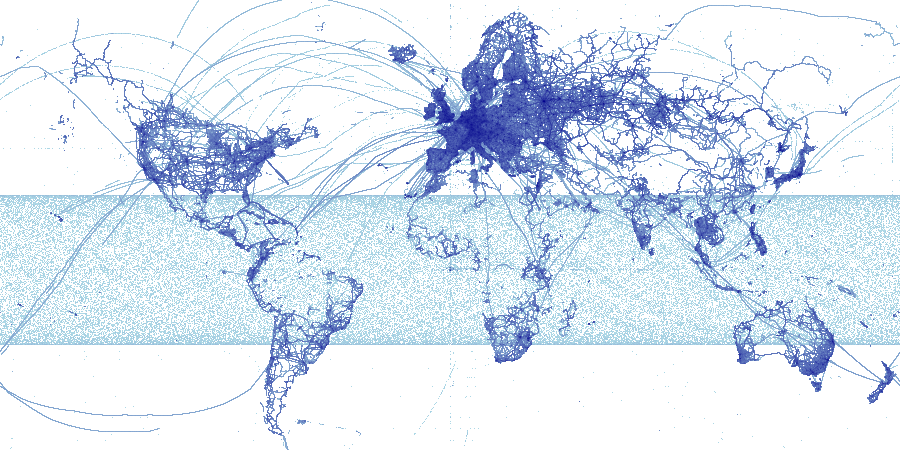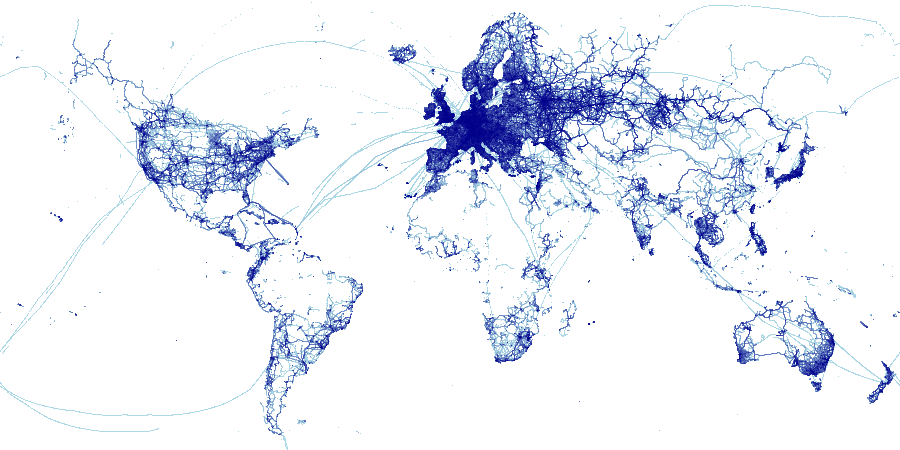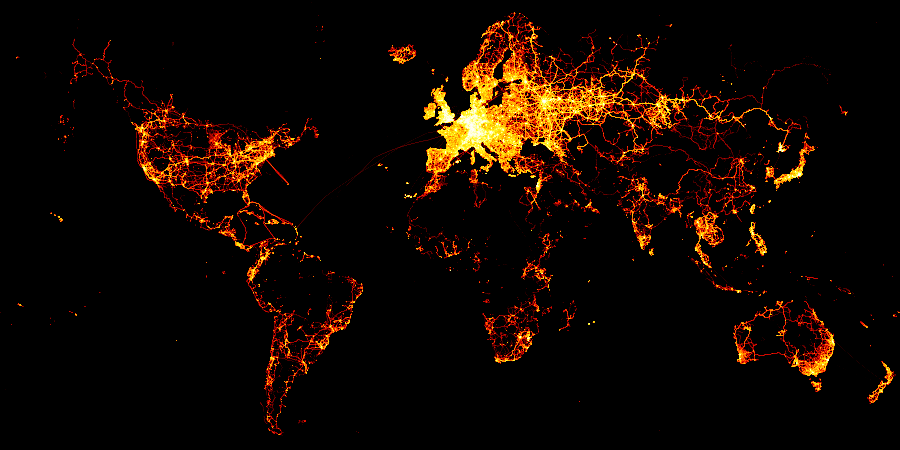# Osm-1billion¶

## Datashading a 1-billion-point Open Street Map database¶

The osm.ipynb example shows how to use Dask's out of core support for working with datasets larger than will fit in memory. Here, we consider the largest dataset that will fit comfortably on a 16GB machine, namely 1 billion x,y points (at 32-bit float resolution). You can download it from our site:

and unzip it into the ./data/ directory, but because of its size it's not downloaded with the other examples by default, and please try to limit the number of times you download it so that we can continue making it available.

NOTE: This dataset is also explorable through the Datashader example dashboard. From inside the examples directory, run: DS_DATASET=osm-1b panel serve --show dashboard.ipynb

The data is taken from Open Street Map's (OSM) bulk GPS point data. The data was collected by OSM contributors' GPS devices, and was provided as a CSV file of latitude,longitude coordinates. The data was downloaded from their website, extracted, converted to use positions in Web Mercator format using datashader.utils.lnglat_to_meters(), sorted using spacial indexing, and then stored in a parquet file for faster disk access. As usual, loading it into memory will be the most time-consuming step, by far:

In :
import datashader as ds

from colorcet import fire

In :
%%time
df = df.persist()

CPU times: user 27.8 s, sys: 19.8 s, total: 47.7 s
Wall time: 19 s

In :
print(len(df))

1000050363

Out:
x y
0 -16274360.0 -17538778.0
1 -16408889.0 -16618700.0
2 -16246231.0 -16106805.0
3 -19098164.0 -14783157.0
4 -17811662.0 -13948767.0

### Aggregation¶

To visualize this data, we first create a canvas to provide pixel-shaped bins in which points can be aggregated, and then aggregate the data to produce a fixed-size aggregate array. For data already in memory, this aggregation is the only expensive step, because it requires reading every point in the dataset. For interactive use we are most interested in the performance for a second aggregation, after all of the components and data are in memory and the user is interacting with a plot dynamically. So let's first do a dry run, which will be slightly slower, then do the full aggregation to show what performance can be expected during interactive use with a billion datapoints:

In :
bound = 20026376.39
bounds = dict(x_range = (-bound, bound), y_range = (int(-bound*0.4), int(bound*0.6)))
plot_width = 900
plot_height = int(plot_width*0.5)

In :
%%time
cvs = ds.Canvas(plot_width=plot_width, plot_height=plot_height, **bounds)
agg2=cvs.points(df, 'x', 'y', ds.count())

CPU times: user 11.9 s, sys: 18.4 s, total: 30.2 s
Wall time: 6.06 s

In :
%%time
cvs = ds.Canvas(plot_width=plot_width, plot_height=plot_height, **bounds)
agg = cvs.points(df, 'x', 'y', ds.count())

CPU times: user 10.3 s, sys: 192 ms, total: 10.5 s
Wall time: 1.98 s


As you can see, once everything has been loaded, aggregating this billion-point dataset takes a couple of seconds on a 16GB Macbook laptop. The remaining steps for display complete in milliseconds, so those times are not shown below.

### Transfer Function¶

To actually see the data, we need to render an image of the aggregate array. Let's map small (but nonzero) counts to light blue, the largest counts to dark blue, and interpolate according to a logarithmic function in between:

In :
tf.shade(agg, cmap=["lightblue", "darkblue"], how='log')

Out:There's some odd, low-count, nearly-uniform noise going on in the tropics. It's worth trying to figure out what that could be, but for now we can filter it out quickly from the aggregated data using the where method from xarray. We'll also switch to the parameter-free histogram equalization method for mapping to colors using various colormaps:

In :
tf.shade(agg.where(agg > 15), cmap=["lightblue", "darkblue"])

Out:In :
tf.set_background(tf.shade(agg.where(agg > 15), cmap=fire),"black")

Out:The result covers the most-populated areas of the globe, with Europe apparently having particularly many OpenStreetMap contributors. The long great-circle paths visible on the white background are presumably flight or boat trips, from devices that log their GPS coordinates more than 15 times during the space of one pixel in this plot (or else they would have been eliminated by the where call).

### Interactive plotting¶

As long as the data fits into RAM on your machine, performance should be high enough to explore the data interactively, with only a brief pause after zooming or panning to a new region of the data space. Performance should be much higher when you are zoomed in, thanks to the spatially sorted data structure used here; if you use a regular Pandas or Dask dataframe it will need to iterate through the entire dataset on each zoom rather than only considering a subset as used here.

In :
import holoviews as hv
from holoviews import opts